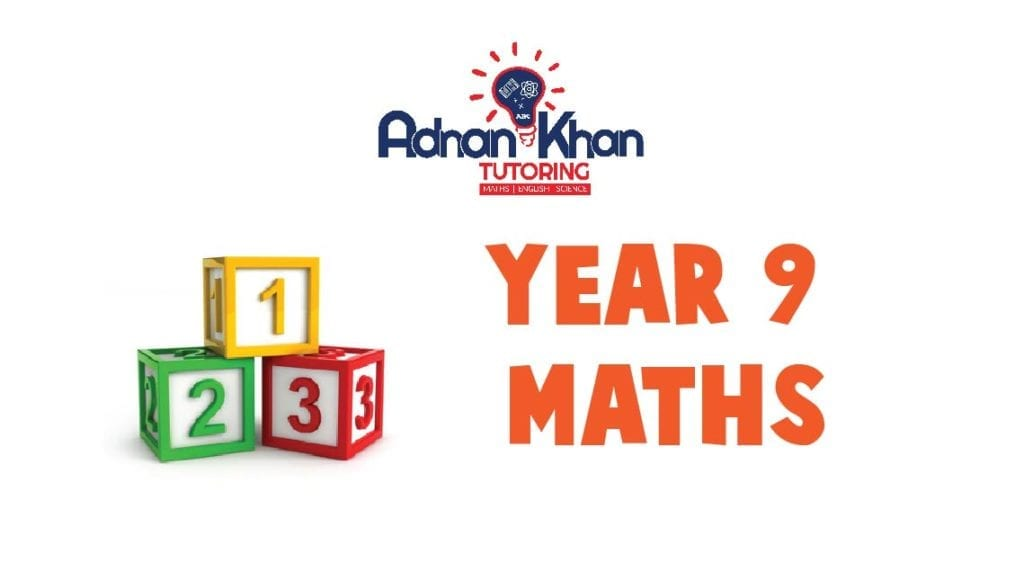# Year 9 Maths Tutors in High Wycombe

Year 9 is one of the important school years. It is crucial as students need to prepare themselves for GCSE years. Math is the most tutored subject in the world because of its complexities and concepts. We have seen parents hiring year 9 Maths tutors in High Wycombe and the UK. It’s good that parents make sure the child gets quality learning with the best possible help. We offer premium online tuition for year 9 maths, covering all lessons included in the National Curriculum. Private tutors for year 9 are also available in High Wycombe, but they are not easily affordable.

Adnan Khan Tutoring offers a comprehensive range of online courses in Year 9 Maths tuition in High Wycombe and the UK. Our hi-tech tutoring platform is user friendly. We provide exclusive year 9 Maths tutoring with qualified online tutors. Our tutors provide regular feedback to parents and check the homework thoroughly at the end of each session. We offer a free trial without any signup obligation. All our courses are affordable.

## What does our Year 9 Maths course include?

Our online year 9 Maths lessons are divided into four sections. The first section is Number Crunching consists of Ordering Numbers and Place Value, Multiplying by 10, 100, dividing by 10, 100, Adding, Subtracting, Adding Decimals, Subtracting Decimals and more complex Maths lessons.

The second section is Shapes and Area, having lessons: Regular Polygons, Symmetry, Quadrilaterals, Families of Triangle, Vertices, Faces and Edges, Perimeter, Areas of Rectangles, Areas of Triangles and more lesson on shapes and areas.

The Handling Data has lessons: Statistical Investigations, Scatter Graphs and Correlation, Time Series Graphs, Two-way Tables, Comparing Two or More Sets of Data and more related lessons.

The Shape, Space and Measures section includes lessons: Angles of Polygons, Angles of Regular Polygons, The Circle and its Parts, Constructing Right-Angled Triangles, Loci and other lessons.

Algebra

• Sequences
• Pattern Spotting
• Functions
• Graphs
• Limits of Sequences
• Equations and Formulae
• Solving Problems Using Equations
• Equations Involving Fractions
• Equations involving x2
• Trail and Improvement
• Graphs Showing Direct Proportion
• Factorisation
• Index Notation with Algebra
• Square and Cube Roots
• Expand and Simplify
• Factorising
• Substitution
• Change of subject
• Graphs of Linear Functions

Number

• Multiplying and Dividing Fractions
• Percentages and Compound Interest
• Reverse Percentages and Percentage Change
• Ratio
• Number between 0 and 1
• Rounding and Estimation
• Powers of 10
• Standard Form
• Bounds
• Multiplying Decimals
• Dividing Decimals
• Recurring Decimals
• Efficient use of a Calculator
• BODMAS
• Solving Problems

Shape, Space and Measures

• Angles of Polygons
• Angles of Regular Polygons
• The Circle and its Parts
• Constructing Right-Angled Triangles
• Loci
• Geometric Reasoning
• Circumference of a Circle
• The Area of a Circle
• Similar Triangles
• Metric Units of Area and Volume
• Volume and Surface Area of Prisms
• Rates of Change
• Enlargements
• Planes of Symmetry
• Map Scales
• Congruent Triangles

Handling Data

• Statistical Investigations
• Scatter Graphs and Correlation
• Time Series Graphs
• Two-way Tables
• Comparing Two or More Sets of Data
• Probability Statements
• Mutually Exclusive Events and Exhaustive Events
• Estimates of Probability
• Revision of Statistical Techniques
• Handling Data Project

## Enrol Now

#### £129.00

per month for 12 total payments
12 months of access

Categories:

Scroll to Top